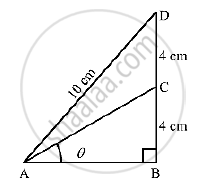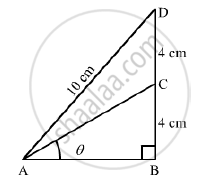# In the Adjoining Figure, ∠B = 90° , ∠Bac = Theta° , Bc = Cd = 4cm and Ad = 10 Cm. Find (I) Sin Theta and (Ii) Costheta - Mathematics

In the adjoining figure, ∠B  = 90° , ∠BAC = theta° , BC = CD = 4cm and AD = 10 cm. find  (i)  sin theta and (ii) costheta#### SolutionIn ΔABD,
Using Pythagoras theorem, we get

AB= sqrt(AD^2-BD^2)

= sqrt(10^2-8^2)

=sqrt(100-64)

=sqrt(36)

=6cm

Again,
In ΔABC,
Using Pythagoras therem, we get

AC= sqrt(AB^2 +BC^2)

=sqrt(6^2+4^2)

=sqrt(36+16)

=sqrt(52)

=2sqrt(13)cm

Now,

(i) sintheta = (BC)/(AC)

=4/(2sqrt(13))

=2/sqrt(13)

=(2 sqrt(13))/13

(ii) cos theta = (AB)/(AC)

= 6/(2sqrt(13))

=3/sqrt(13)

=(3sqrt(13))/13

Concept: Trigonometric Ratios and Its Reciprocal
Is there an error in this question or solution?

#### APPEARS IN

RS Aggarwal Secondary School Class 10 Maths
Chapter 5 Trigonometric Ratios
Q 24